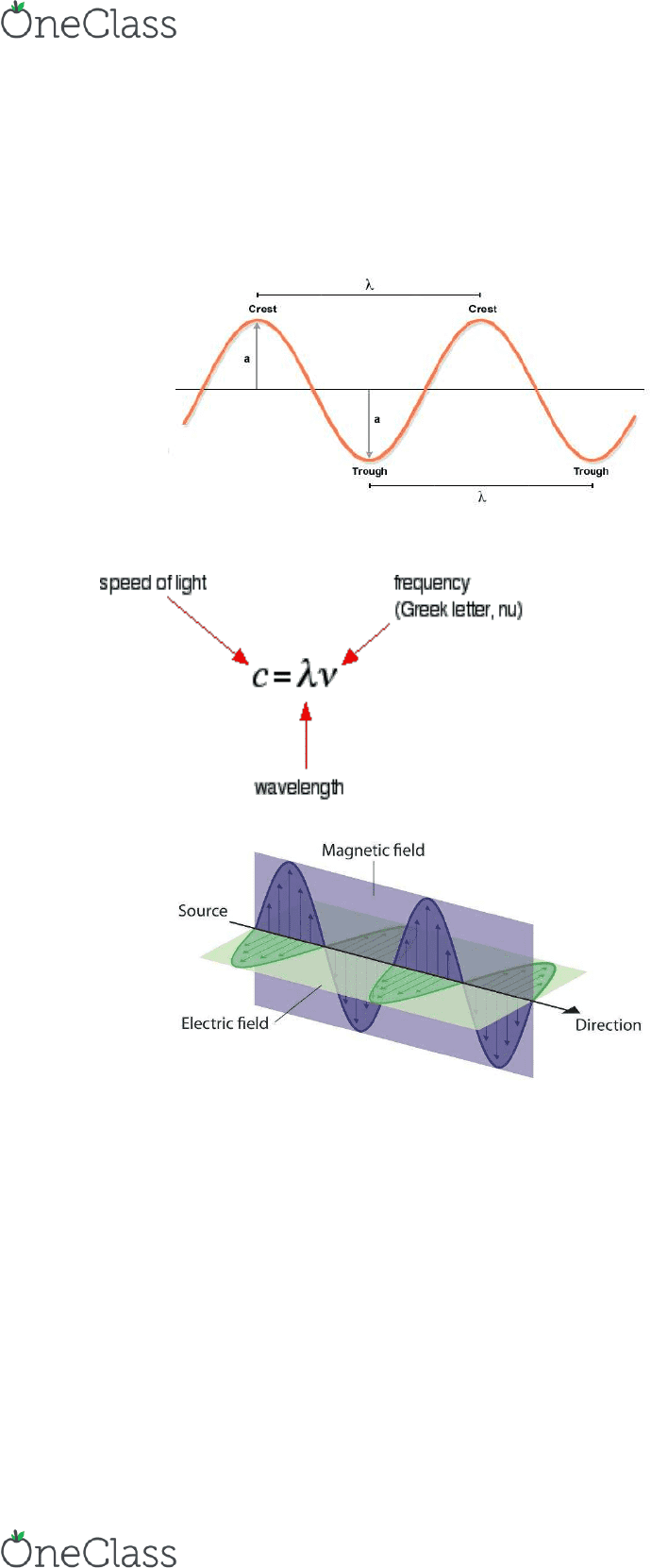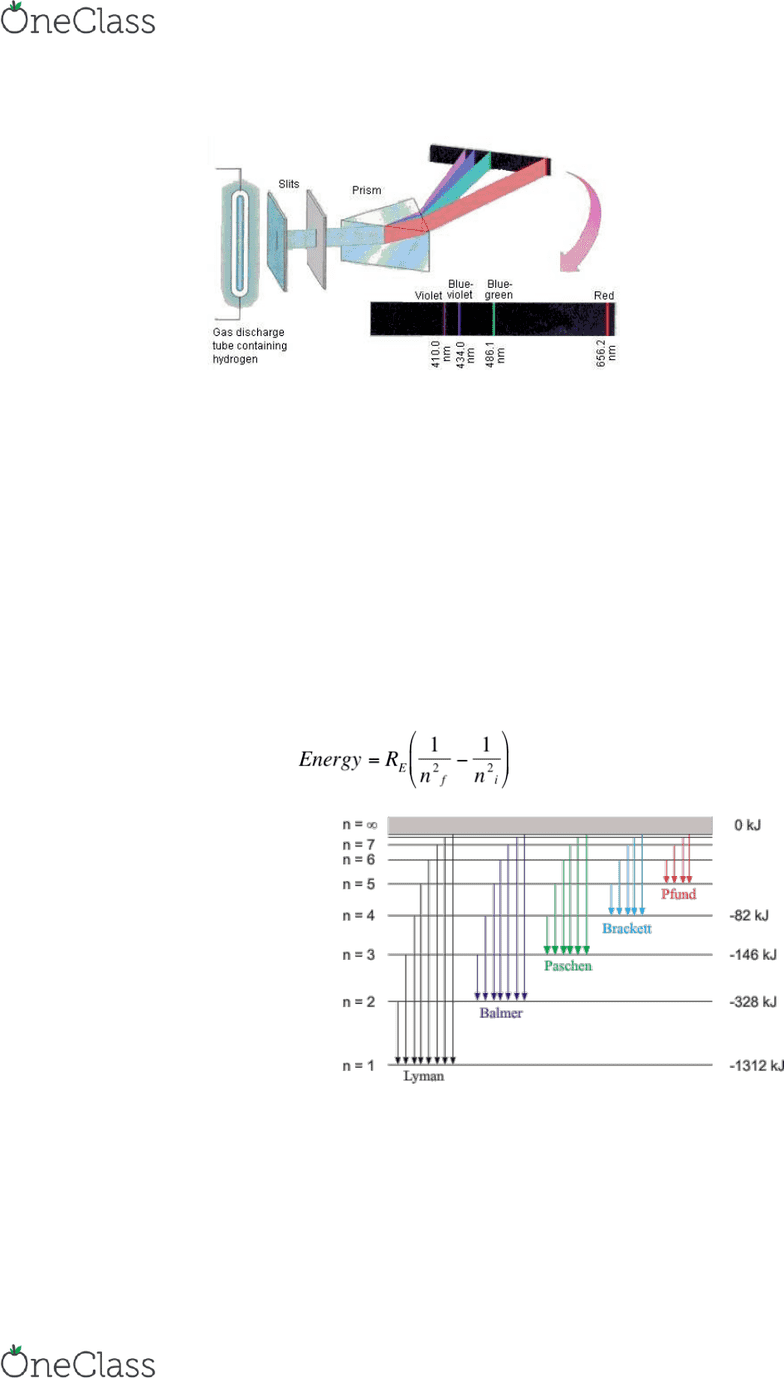Textbook Notes (280,000)
US (110,000)
CHM (9)
Chapter 7

# CHM 111 Chapter Notes - Chapter 7: Gas-Filled Tube, Uncertainty Principle, Atomic Orbital

This preview shows page 1. to view the full 5 pages of the document.CHAPTER 7
Waves: vibrating disturbance by which energy is transmitted
Wavelength () - distance between identical points on successive waves
Frequency () - # waves that can pass thru a point in 1 second - unit = Hertz (Hz) (1/s)
Amplitude - vertical distance from middle of a wave to peak/trough
Electromagnetic radiation: has electric field component and magnetic field component
o Emission and transmission of energy in the form of electromagnetic waves
o c = speed of light = speed of electromagnetic waves = 3.00E8 m/s
o of waves is normally given in nm (nanometers) (10E-9m)
o Highest frequency: rays (gamma)
Planck’s Quantum Theory
o Quantum: smallest quantity of energy that can be omitted/absorbed in the form of
o E=h*=h*c/ → energy of single quantum
h = Planck’s constant = 6.626E-34 Js = 6.626E-34 m2 kg/s
The Photoelectric Effect
o Electrons are ejected from the surface of certain metals exposed to light above
“threshold frequency”
o Photons: particles of light
find more resources at oneclass.com
find more resources at oneclass.com

Unlock to view full version

Only page 1 are available for preview. Some parts have been intentionally blurred.o Light behaves like particles and waves (aka particle-wave duality)
o E = h*v = KE + W
Bohr’s Theory of the Hydrogen Atom
o Emission spectra - continuous or line spectra of radiation emitted by substances
o Line Spectra: light emission only at specific
Units: Angstroms
1 = 10E-10 m
o Bohr attributed emission of radiation by energized H atom to electron dropping from
higher-energy allowed orbit to a lower one & emitting quantum of energy (photon) in
the form of light
En = -RH*(1/n2)
o RH = Rydberg constant = 2.18 E -18 J
o n = principal quantum number
o E = 0 → energy of free electron - as n decreases, En gets more negative
o n=1 → most negative value → “ground state” - lowest energy state of a system
o n=2,3… “excited state” - higher in energy than ground state
o n increases, orbit radius increases rapidly
o Radiant energy emitted in form of photon when electron moves from high to low
energy state
E = Ef - Ei
Dual nature of the electron
o Electron bound to nucleus behaves like a standing wave
o Nodes have amplitude of 0
o 2r=n
o =h/mu → wavelength = Planck’s constant over mass times velocity (associated w/
moving particles)
Heisenberg Uncertainty Principle
o It is impossible to know both the momentum (p, m*u) and the position (x) of a
particle simultaneously with certainty
find more resources at oneclass.com
find more resources at oneclass.com